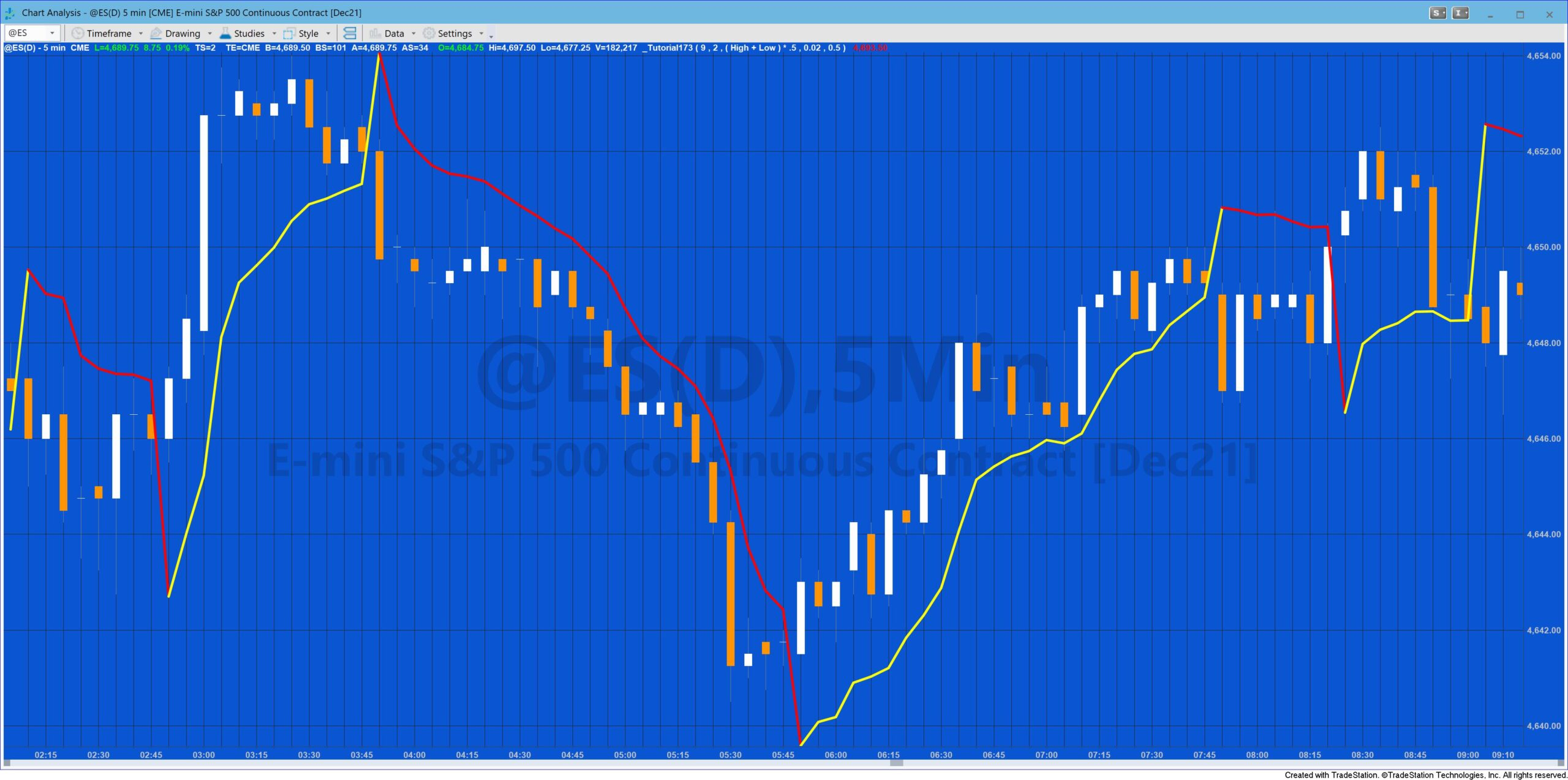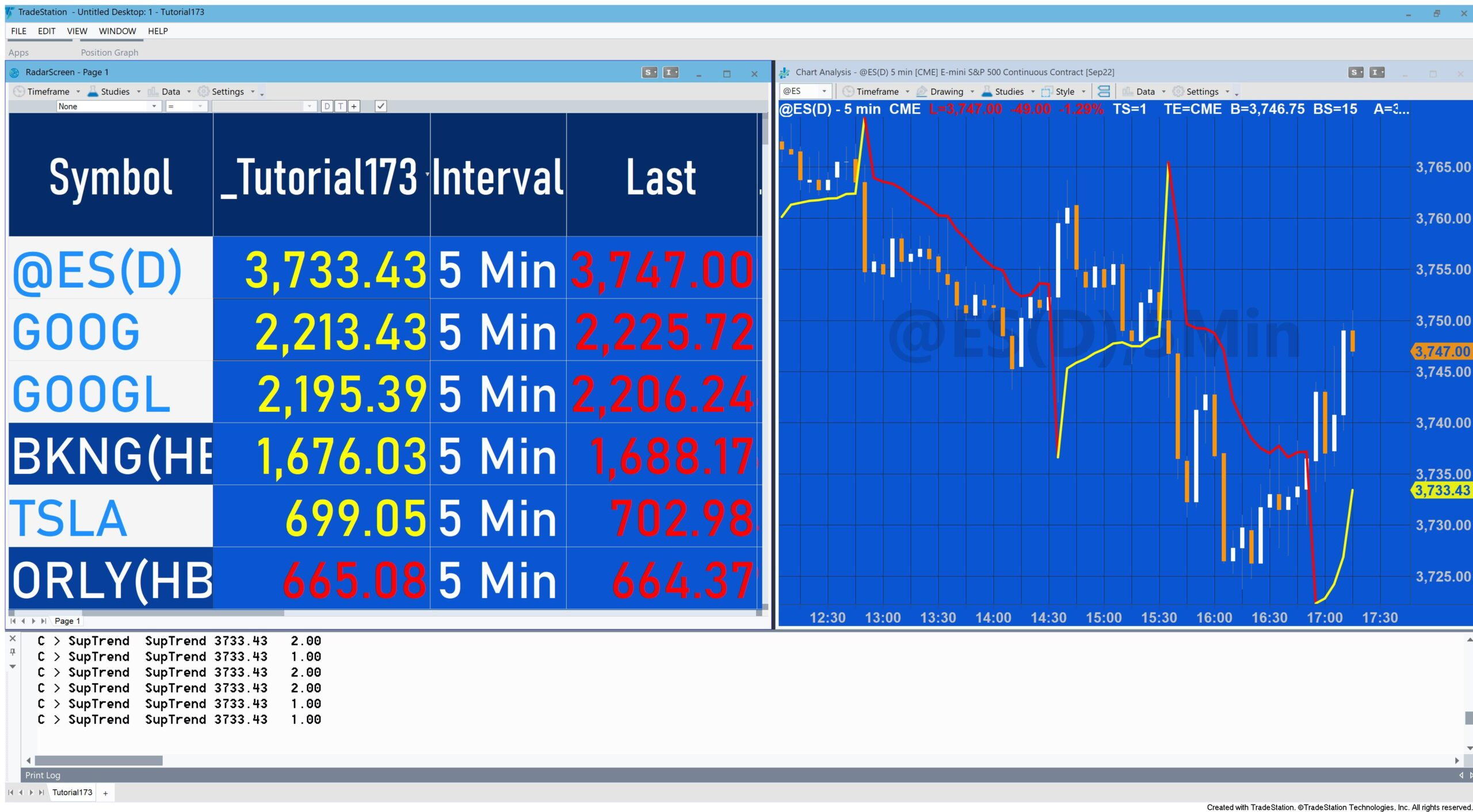# Tutorial 173 | Markplex SuperTrend

Tutorial 173 | SuperTrend indicator is a stop and reverse (SAR) indicator that trails price action. This implementation calculates the Average True Range (ATR) of the average price using the exponential moving average. A user input multiple of the ATR is added to or subtracted from the average price to give Upper and Lwr
respectively, depending on the direction. Direction is determined by the Close of a bar relative to the value of the SuperTrend, Upper and Lwr using the following code:

`If C > SupTrend then BeginDir = 1;PlotColor = Yellow;//Print( " C > ST c ",c," SupTrend ", SupTrend," ",BarColor);End else if C < SupTrend then BeginDir = -1;PlotColor = Red;// Print( "C <= ST "," ",BarColor );End;`

When the direction is up (Dir = 1), the SupTrend value can only increase. When the dirction is down, the SupTrend can only decrease.

There are many different interpretations of the so-called SuperTrend indicator. In this Markplex version the SuperTrend value is also tightened (i.e. moved closer to price) by using an acceleration factor which increases incrementally each bar by user input: AFStep up to a a maximum value of user input: MaxAF.

The indicator works best in trending markets but gets ‘whipsawed’ in other markets.Tutorial 173 can be used with TradeStation OR with MultiCharts.
See a video walk through of tutorial 173.

If you want to save yourself some typing,  the tutorial 173 code is available for immediate download for \$19.95 by clicking the following button. You can pay using PayPal or credit card. The program works with TradeStation 9.5 and 10.

See tutorial 43 which demonstrates how to import an ELD into TradeStation.

### Using tutorial 173 with RadarScreen

The above video demonstrates how to set up Tutorial 173 (Markplex SuperTrend) in TradeStation RadarScreen. This involves making sure that “load additional data for accumulative calculations” is selected and an appropriate number of bars is entered.

“Load additional data” is required for calculations that use the value of the calculation the previous bar to give the value for the current bar. Tutorial 173 uses the exponential moving average as part of its calculation. If you open TradeStation’s XAverage function, you will see the calculation includes:

`XAverage = XAverage + SmoothingFactor * ( Price - XAverage );`

In other words, the exponential moving average for the current bar is equal to the exponential moving average for the previous bar plus the difference between the price of the current bar and the exponential moving average of the previous bar multiplied by a smoothing factor.

TradeStation recommend that the number of additional bars is equal to 4.5 multiplied by the Length input. In this video I estimated this to be 50.